Simulation of the Universe

By

Neil J Boucher December 17, 2003

Synopsis

The article looks at what it would take to simulate the universe and what such a universe might look like.  Could we tell if we were living in such a universe??

The general conclusion is that it is decidedly possible to simulate the universe, provided the universe is quantized and finite, but that it would fail the Occam’s razor test in that it would not be the simplest way to build a universe.

Here we consider what would be necessary to completely simulate the known universe.  We find that it is not something that can be achieved from within the universe.

The are about 10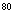particles in the universe and if we allow each to have 10 properties (which will include location, momentum, spin, charge, mass) then we have to store 10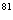pieces of information.  However looking at this a bit more closely it becomes a bit more complex.  If, in the real world, momentum for example can take on any value then it can take on values that include irrational values.  Even to store one such value we would need infinite storage capacity.  Therefore to be computable the world must be quantized! It also follows that all information about the universe and its components must be quantized if we are to even consider computerizing it. This includes the scale of the universe and so precludes an infinite universe. The number of bits for storage of information (as distinct from pieces of information, as one velocity for example might require 40 bits to store it) will depend on the quantizing scale.

Since a top end PC might have 500 gigs of memory (5 x 10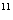bytes) we fall a long way short and cannot even consider it a candidate.  In fact we would need to do something rather clever to store enough information because we would need, as a minimum, all the particles in the universe just to store the information if we did it the way a PC does. This holds true even if we could find some way to optimize the use of those particles (your PC might use 1000’s of atoms, or 100,000’s of particles to store 1 bit of information).

So if we were to ask the question can we precisely model the known universe, from within the known universe, the answer must be NO.  We can only model much simpler and smaller universes than the one that we occupy.

This eliminates the multiple mirror image computational model; that is, we cannot have universes that contain computers that in turn spawn new universes as models, unless those models are significantly smaller and less complex than the original universe that originated the model.

In an article for Nature, June 2002, Seth Lloyd of MIT in Cambridge gives the memory capacity of a universe simulating computer at 10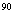bits and a CPU that would have manipulated 10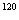bits of information in the time since the big bang.  His estimate is based on the energy equivalent of the universe, but can be seen to be consistent with the number of particles contained within.

The God Problem

In primitive societies man looked about and saw that all was too complicated to explain and so he invented a god that “explained it all”. There is however a problem with this approach.  Suppose you find a watch on a deserted island, and you can’t understand how it came to be, so you conceive the watch maker.  Now the watch maker is more complex that the watch so you conceive a god. The logical process of this thinking is that there is a god of gods and so on.  Each new god more complex than the one before. This really leads nowhere.  To say that one of the god’s “always was, and did not need a creator” is simply avoiding the question and does not explain anything.  An explanation must simply the situation not add complexity to it.

The computational model of the universe has to face its own god problem.  The computer that runs the simulation is necessarily more complex, contains more information and is “god-like” compared to the universe itself.  You have not solved anything at all by postulating a computational universe, but have replaced a difficult problem about the complexity of the universe, with a yet more complex machine to run it all in.

The Quantum Mechanical Problem

Many have looked at quantum mechanics as a “proof” of a computational universe.  This approach suffers from the same sort of problem as the God concept.  Firstly and fore mostly no one has any idea what is going on at the quantum mechanical level.  Quantum mechanics is not a description of quantum mechanical reality, but a mathematical construct that mimics the outward behavior of the quantum world.

To illustrate this lets assume someone has somehow managed to model the share market.  Lets say that the model is accurate to +/-5%  (this in itself would be an incredible achievement).  Now such a model can easily be seen as nothing more than a model, and it does not tell us anything about you or me as investors, but simply mathematically predicts the herd behavior.  It is also not necessarily unique.  Other models based on different premises might give equally good results.  A good working stock market model will most likely be a substitute for real knowledge rather than evidence of complete understanding.

The masters of quantum mechanics are aware that their mathematics is a working model and not something based on a deeper understanding. The fact that the current quantum mechanical model is inconsistent with Relativity suggests that it is incomplete. Its high level of agreement with experiment to date shows it to be a very good model, but there is reason to believe that some day researchers will find discrepancies between the theory and measurement.  After all, the model is known to be incomplete and this must at some time show up as a disagreement with measurements if they are precise enough.

Now we see the problem.  The mathematics of quantum mechanics is not reality based, but is a mathematical construct that mimics the reality as seen in the laboratory.  One should not be surprised therefore that the mathematics looks like a model because that is all it is.

So the argument that quantum mechanical behavior looks computational is circuitous. Quantum mechanics as we know it, is a model of reality and nothing more.  It has never claimed to be otherwise.  We cannot directly infer anything about the real nature of the universe from quantum mechanics.

A Computer for a Computational Universe

As we have seen the universe that we occupy cannot be computed from within the universe itself.  That is if the universe were computational, the stuff that the computer itself is made of is not of this universe and has no need to be related to the universe in any way.  To clarify a computer that flies the space shuttle or one that makes cars will be of the same kind and may even have the same operating system. The computers may even happen to be identical.  However the stuff that the computer is made of is totally independent of its function and the computer makers need not know in advance that application that the computer is intended for.

So a computer that simulates the known universe need not, and probably would not be made of electrons, protons and photons etc. These particles should then be regarded as  simulated and their forms (properties) likewise can be simple mathematical constructs.

It is unlikely that the computer would be made of the same sort of stuff that it is simulating. Likewise the constants of the known universe (gravitational constant, the ratio of the mass of a proton to and electron and such) can be simple mathematical starting points.  Other limitations like the speed of light need not be applicable in any way to the computer itself.  This gives us the possibility of allowing that the computer simulation has looked at many possibly worlds where many possible particle properties have been tried and the successful models are the ones that are left running.

Consciousness

A lot of people seem to have a problem with consciousness, and I am not sure why this is.  The brain is a massively parallel computer that has many conflicting processes.  For example the fight or flight response is really a fight and flight response.  The adrenalin prepares us for both and the brain considers both; not so much sequentially but in parallel.  On this issue and many others, the left and right hand side of the brain will come to totally conflicting conclusions.

Therefore a higher function of the brain has to be to manage all these inputs and decide on a course of action.  It seems straight forward enough to allow that this management center would start as a fairly basic bit of brain hardware, but as the brain evolves the demands on the management system would rapidly increase.  The management center in the human brain would be called on to make many varied and complex decisions.

Consciousness in this light is the evolution of the management function from one that simply resolves conflicting “reports” from other brain centers to one whereby the management system can reflect on its own performance (that is it begins to manage itself).  The management system is now not only aware of the other brain functional centers but becomes aware of itself.  This argument is consistent with the point of view that all brains except the most simple would have evolved some form of self consciousness and that the level of consciousness would increase with the brain complexity.

Consciousness in this view has a purpose.  A management system that does not review its own performance would be doomed to repeat the mistakes of the past.  However one that does have a review process, can allow the subordinate centers to show a degree of folly and ignore it.

Suppose the management center were not self reviewing and was simply an automatic “judge”.  It simply weighs the inputs from all the competing brain sections and makes a decision.  In order to avoid repeating mistakes then since the management is not self reviewing, the subordinate centers need to get feedback on the result of there advice and to modify their advice for the next similar event accordingly.  While this may happen in some instances, there is a lot of evidence from clinical studies that the subordinate parts of the brain do not have good feedback paths.  In split brain experiments one part of the brain will consistently deny a truth known to the other part of the brain.  Conflict over something as simple as whether or not the person likes a certain food can exist between the two halves of the brain.  Another instance of this is a person with brain damage who could recognize his mother readily when she called in by phone, but would insist that she was an imposter when she appeared in person.

From the experiments above (and others) I think we can conclude that the subordinate parts of the brain are only minimally conscious and are not especially good at communicating to each other.  The management system is thus the bit that becomes self aware.  As a caution here, when speaking of parts of the brain the meaning should be taken as “functional parts of the brain”.  It is not suggested that the brain is physically partitioned into a conscious and an unconscious part, but rather that this partition is functional.

So to be truly efficient the brain needs to be self-appraising on some level and it appears that this is the highest level.  If consciousness requires a considerable computing overhead it would make sense to centrally locate it.  The importance of this is that were the consciousness to be distributed it would not need to be particularly “strong” because at each place that it occurred it would be specialized.  By centralizing consciousness we are giving consciousness (which is merely initially at least the ability for self-performance evaluation) a chance to evolve into something very powerful indeed.

Because the management center makes its decisions in a different way to the rest of the brain it would be important when weighing the inputs that it was aware that its own input (essentially memories of  its past decisions) are of a different kind and need to be treated differently to the subordinate inputs.  Thus from an early evolutionary stage it would be an advantage to distinguish its own inputs from the others.  It is already starting to become self aware!

Really therefore consciousness is not of itself a problem for a computational universe.  The computer needs to be massively parallel with a management system that can itself evolve.

Quantum Computers

Much has been made of the potentially staggering computing power of a quantum computer.  However with today’s limited knowledge of quantum computers there is no certainty at all that large quantum computers could ever be built.  The problem is that the quantum nature only reveals itself at very small scales.  Once a large number of particles are assembled the quantum nature fades away to make way for classical behavior. The result of this is that a 10 particle quantum computer is still a thing of the future and that its capabilities, even when built, would not match that of a \$3.00 handheld calculator.  Current theory is not capable of predicting upper limits to the size of a quantum computer, simply because it is not understood what it is that governs the transition to classical behavior.

We also need to be careful to distinguish computational power with storage capabilities.  Potentially, the quantum computer might be the ultimate number cruncher, but it does not offer an equivalent boost in storage capabilities. Additionally what gives the quantum computer its power is its massive parallel computing power; the downside however is that once we extract one of the solutions we lose the others.

Quantum computers could come in handy however for generating true randomness; which is something that a macro computer is unable to do.  The quantum computer need not be especially powerful to do this and such a device is within the feasibility of today’s technology.

Non Computable Things

Many things are not computable.  For example irrational numbers like pi cannot be represented digitally on a computer. It is approximately 3.14159 or more closely 3.14159 26535 89793 23846 26433 83279 50288 41971 69399 37511 (the value to 50 decimal places), but no matter how many digits we calculate it to we will never have it precisely. As the true value of pi is infinite in length then it would require an infinite storage capacity to hold it.  However in a quantized world, this is not a problem as there is a “smallest” dimension of space.  Therefore the concept of a perfect circle is a fiction and any real circle will in fact be a polygon with a finite number of sides. In such a world pi is merely and abstraction.

So this is not an argument for or against a computable universe, rather it is one against the fiction of the mathematical concept of pi.  In a computable universe the value of pi, like all other values would be truncated to a finite precision.

The Virtually Non-computable Real World

Mathematicians and some physicists have convinced themselves and many others that the world is essentially a mathematically dominated domain.  Mathematicians only study problems that lead to neat mathematical solutions and for them it is a case of not seeing the woods for the trees.  Physicist proceed to eliminate the clutter (the real world) and concentrate on the essence (the part of the system that they are studying, isolated from outside influences).  So studying one atom in isolation might lead to some rather neat mathematics. Two atoms are decidedly messy and anything more is quite frightful.

A good example of the consequences of this is the study of ballistics.  The path of a ball (lets say a cannon ball) through a vacuum under the influence of gravity that does not go too high, has a neat mathematical solution.  It is so neat and beloved by the physicist that even today school children are taught to calculate the path of a cannon ball fired through a vacuum. The fact that probably no cannon has ever been fired in a vacuum is not mentioned.  Most texts dismiss the atmosphere as something that will cause some drag on the cannon ball but for the purposes of the study can be ignored.  The truth is that allowing for the drag is very messy and the neat mathematics dissolves when account is taken of this parameter.  Without the drag the vertical and horizontal motions can be regarded as occurring independently and out pops a simple solution.  Allow for the drag and this independence disappears.  The motions become correlated and no neat solution is possible (the solution requires a computer to do a lot of approximate calculations over many small paths).  But here’s the punch line…the error that results from ignoring the drag of the air is about 50% (the actual value varies according to the drag coefficient of the projectile and its speed).  This huge error is conveniently ignored! Even the famous conclusion that the angle of launch for maximum range is 45 degrees turns out to be wrong when the drag it taken into account (41 degrees or thereabouts is more like it).

A related example is the many body problem.  It is relatively easy to model mathematically two bodies that are isolated from the real world that orbit around one another.  Make it three (for example as in the earth, moon sun) and the task becomes most formidable.  Go to four or more and it is virtually impossible. And yet most solar systems are more complex than a four body situation  particularly if the asteroids are included.

Another seeming simple problem is the solution to a detector circuit as seen in figure 1 below. In fact this circuit could have come out of a high school physics book.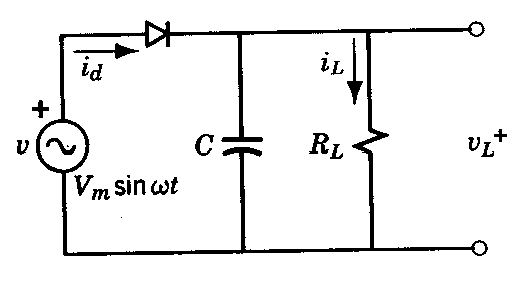The circuit consists of a signal source, a diode and a load with a filtering capacitor.  If enough simplifying assumptions are made it is relatively easy to extract mathematical solutions for this circuit.  However if we look into the situation a bit further we find the situation is definitely too complex for high school consideration.

Lets accept that the diode equation is I=Io.e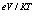Where e= the charge of an electron

V= the voltage across the diode

K=Boltzmann’s constant

T= the absolute temperature.

Io= the reverse leakage current of the diode (a constant)

I= the current through the diode.

which of itself is a simplification of the diode behavior so we are already filtering some of the clutter already. We can simplify this even more by noting the e/kT=40 (approximmately) so we can model the diode as

I=Io.e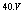eq 1

Lets ignore the capacitor for now (more clutter that makes the problem harder) and just consider the load resistance.

The voltage loop around the circuit would give us

Vs=V+I.R   eq 2

where R is the load resistance

Vs is the generator voltage (instantaneous value).

So here we have a fairly simply pair of equations that define the circuit mathematically. However the simplicity is deceptive.  We can conclude that

Vs=V+ Io.e.R   but to solve this for V is anything but easy.

These are but a few examples of the enormous difficulty of actually using mathematics to model the real world.  Most real world problems are too complex to yield to mathematics.  This is not to say that all is lost.  We can use approximate numerical methods to model all of the above examples to a very high degree of accuracy.  However the solutions are not neat mathematical ones but involve iterative methods that start with a guess and then home in on the solution.  Numerical methods are widely used and consume much computer time but they are evidence of how poorly the real world conforms to mathematics.

In summary, if you simplify the problems by removing the real world clutter, you should not be surprised that you get neat mathematical solutions, because that was why you removed the clutter in the first place.

Chaos

Chaos theory tells us that the outcome of any chaotic event is highly dependent on the initial state.  If the initial state itself is uncertain (as it must be in quantum mechanics) then the outcome is unpredictable and any initial uncertainty will multiply over time. If computers were to mimic nature they would need to quantize everything. Quantizing errors are additive and in a chaotic system even small errors can lead to very different outcomes for the same original state.

However such graininess exists in the real world. A world system that brings the weather into being can be resolved down to molecular parts and at this level the patterns would differ from those of a continuum.  So the real world becomes digital in that sense. A computer with sufficient resolution would not be distinguishable from the real thing.

Conclusion

If the universe is a simulation of if it is simulable then the following is true.

• There is no place in such a universe for irrational numbers like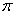and e and any equation containing these numbers will be an approximation.
• In a computed or computable universe the equations of physics will be digital.  That is the only permitted solution will be integer multiples of the small units (of space, .time, mass etc).
• The universe and everything in it will be finite.  This would apply also to quantum states
• A simulated universe made of the same stuff as the universe would be inefficient and we should expect that such a computer would be made of other “stuff”, which we can call computrons. Computrons would not be subject to the laws of the known universe.

As an afterthought on decoherence, the computability requirement would leave its mark on this too.  Because computable quantum states would need to be finite in number, then so too would entangled ones.  Assuming that the total permitted number of states (the finite number) is a constant, entangled quantum states would become more and more restrictive.  The most improbable states would become increasingly improbable (there are more of these states so that if the total is fixed the more improbable states would be the big losers).  So a collection of quantum objects would not suddenly decohere as is the current expectation, but they would slowly become less and less quantum-like as the number of entangled objects increased.

Decoherence by interaction with a non quantum object would simply be the result of an entanglement of a quantum object with one that has massively restricted options (the non-quantum object) When the entanglement occurs the quantum object becomes part of the “non-quantum” object and the restriction that the total number of  probabilities be a constant would rob the quantum object of its quantum nature.

Thus a computable world would be detectable.  The finite-ness of it would give it away.